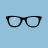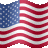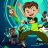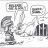# Trend Momentum Divergence Indicator for ThinkorSwim#### BenTen

Staff
VIP
Warehouse### thinkScript Code

Rich (BB code):
``````#That will show mostly price within it's range compared to the indicator within it's range. Since the indicator is bound and price is free to run at any angle it please for as long is it damn well likes, it more or less will only allow you too see price against your indicator. If I was going to trade it on shorter timeframes, I would use this version

#

# Yet Another Divergence Script - Trend Momentum Divergence

# Nube 6.5.18

#hint: Usess linear of price scaled to RSI as an indicator of trend

declare lower;

input Length     = 14;

input OverBought = 70;

input OverSold   = 30;

input Cloud      = yes;

def na = Double.NaN;

def indicator  = RSI(Length = length);

script scale {

input c = 0;

input Min = 0;

input Max = 1;

def hh = HighestAll(c);

def ll = LowestAll(c);

plot Range = (((Max - Min) * (c - ll)) /  (hh - ll)) + Min;

}

def sPrice = scale(close, Lowest(indicator, length), Highest(indicator, length));

plot

OB  = if !IsNaN(close) then OverBought else na;

OB.SetDefaultColor(Color.Light_Red);

plot

OS  = if !IsNaN(close) then OverSold else na;

OS.SetDefaultColor(Color.Light_Green);

plot

RSI = indicator;

RSI.DefineColor("Over Bought", Color.Red);

RSI.DefineColor("Over Sold", Color.Green);

RSI.DefineColor("Typical", Color.White);

RSI.AssignValueColor(

if    RSI > OB

then  RSI.color("Over Bought")

else  if   RSI < overSold

then RSI.color("Over Sold")

else RSI.color("Typical")

);

plot

priceTrend = Inertia(sPrice, length);

priceTrend.DefineColor("Price Trend Down", CreateColor(150,50,50));

priceTrend.DefineColor("Price Trend Up", CreateColor(50,150,50));

priceTrend.AssignValueColor(if   priceTrend < priceTrend

then priceTrend.Color("Price Trend Down")

else priceTrend.Color("Price Trend Up"));

CreateColor(50,150,50), CreateColor(150,50,50));

# f/ Trend Momentum Divergence``````Questions 12Indicators 1Custom 9Indicators 145